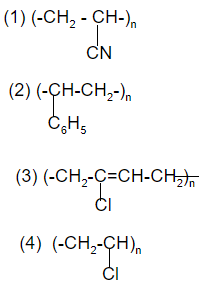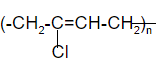# JEE Main Polymer Previous Year Questions with Solutions

Polymer previous year questions and solutions are given in this article. A polymer is a large molecule or a macromolecule which essentially is a combination of many sub units. These can be found all around us. From the strand of our DNA which is a naturally occurring biopolymer to polypropylene which is used throughout the world as plastic. These can be naturally found in plants and animals (natural polymers) or can be man-made (synthetic polymers). These have a number of unique physical and chemical properties due to which they find usage in everyday life.

Polymers are all created by the process of polymerization wherein their constituent elements called monomers, are reacted together to form polymer chains i.e 3-dimensional networks forming the polymer bonds. The important topics include classification, structure, properties, molecular mass and uses of polymers. This article helps students to revise the previous year JEE questions and score higher marks. BYJU’S provide solutions created by our subject experts. Students can easily download these solutions for free.

## JEE Main Previous Year Solved Questions on Polymer

1. Polymer formation from monomers starts by

(1) condensation reaction between monomers

(2) coordinate reaction between monomers

(3) conversion of monomer to monomer ions by protons •

(4) hydrolysis of monomers

Solution:

Polymerisation starts either by addition or condensation reactions between monomers.

Hence option (1) is the answer.

2. Which of the following is a polyamide?

(1) Teflon

(2) Nylon – 6,6

(3) Terylene

(4) Bakelite

Solution:

Hence option (2) is the answer.

3. Which of the following is fully fluorinated polymer?

(1) Neoprene

(2) Teflon

(3) Thiokol

(4) PVC

Solution:

Teflon is Polytetrafluoroethene. So teflon is a fully fluorinated polymer.

Hence option (2) is the answer.

4. Bakelite is obtained from phenol by reacting with

(1) (CH2OH)2

(2) CH3CHO

(3) CH3COCH3

(4) HCHO

Solution:

Bakelite is obtained from phenol by reacting with formaldehyde HCHO.

Hence option (4) is the answer.

5. The polymer containing strong intermolecular forces e.g. hydrogen bonding, is

(1) teflon

(2) nylon 6,6

(3) polystyrene

(4) natural rubber

Solution:

Nylon 6,6 is a polymer of adipic acid and hexamethyline diamine. It has strong intermolecular forces.

Hence option (2) is the answer.

6. Which one is classified as a condensation polymer?

(1) Acrylonitrile

(2) Dacron

(3) Neoprene

(4) Teflon

Solution:

Dacron is a condensation polymer. Teflon, neoprene and acrylonitrile are addition polymers.

Hence option (2) is the answer.

7. Which polymer is used in the manufacture of paints and lacquers?

(1) Bakelite

(2) Glyptal

(3) Polypropene

(4) Poly vinyl chloride

Solution:

Glyptal is used in the manufacture of paints and lacquers.

Hence option (2) is the answer.

8. The species which can best serve as an initiator for the cationic polymerization is

(1) LiAlH4

(2) HNO3

(3) AlCl3

(4) BuLi

Solution:

AlCl3 can serve as an initiator for cationic polymerization.

Hence option (3) is the answer.

(1) Polyester polymer

(2) Polyamide polymer

(3) Polyethylene polymer

(4) Polyvinyl polymer

Solution:

Nylon is a polyamide. It is made from reacting carbon-based chemicals found in coal and petroleum in a high-pressure, heated environment.

Hence option (2) is the answer.

10. Which one of the following structures represents the neoprene polymer:-Solution:

Neoprene is a polymer of chloroprene.Hence option (3) is the answer.

11. The formation of which of the following polymers involves hydrolysis reaction?

(1) Nylon 6

(2) Bakelite

(3) Nylon 6, 6

(4) Terylene

Solution:

The formation of nylon -6 involves the hydrolysis of caprolactum which is a monomer.

Hence option (1) is the answer.

12. Among the following substituted silanes the one which will give rise to cross linked silicone polymer on hydrolysis is

(1) R4Si

(2) RSiCl3

(3) R2SiCl2

(4) R3SiCl

Solution:

RSiCl3 + H2O → RSi(OH)3 + HCl

Trichlorosilane is used in the production of purified cross-linked silicon polymer. It decomposes in water to produce a silicone polymer while giving off HCl.

Hence option (2) is the answer.

13. Formation of Bakellite follows:

(1) Electrophilic substitution followed by condensation

(2) Nucleophilic addition followed by dehydration.

(3) Electrophilic addition followed by dehydration

(4) Hydration followed by condensation

Solution:

Formation of Bakellite follows electrophilic substitution followed by condensation.

Hence option (1) is the answer.

14. Poly-β-hydroxybutyrate-co-β-hydroxyvalerate (PHBV) is a copolymer of

(1) 2- hydroxybutanoic acid and 3-hydroxypentanoic acid.

(2) 3- hydroxybutanoic acid and 3-hydroxypentanoic acid.

(3) 2- hydroxybutanoic acid and 2-hydroxypentanoic acid.

(4) 3- hydroxybutanoic acid and 4-hydroxypentanoic acid.

Solution:

PHBV is a copolymer of 3- hydroxybutanoic acid and 3-hydroxypentanoic acid.

Hence option (2) is the answer.

15. The two monomers for the synthesis of Nylon 6,6 are

(1) HOOC(CH2)6COOH, H2N(CH2)6NH2

(2) HOOC(CH2)6COOH, H2N(CH2)4NH2

(3) HOOC(CH2)4COOH, H2N(CH2)6NH2

(4) HOOC(CH2)4COOH, H2N(CH2)4NH2

Solution:

Monomers of Nylon 6,6 are adipic acid and hexamethylene diamine.

Hence option (3) is the answer.